RUSSIAN JOURNAL OF EARTH SCIENCES, VOL. 16, ES4001, doi:10.2205/2016ES000571, 2016

# Analytical information system for control and spectral analysis of geomagnetic field and space weather parameters

A. V. Vorobev, G. R. Vorobeva

Ufa State Aviation Technical University, Ufa, Russia

### Abstract

Long-term experimental researches in various spheres prove an influence of space weather, geomagnetic field, its variations and anomalies on systems and objects of various origins. The estimation of the influence requires an effective approach to analyze the principles of distribution of geomagnetic field parameters on the Earth's surface, its subsoil and in circumterrestrial space. It is a complicated problem to be solved and it is mostly concerned with monitoring, analytical control and visualization of parameters of space weather, geomagnetic field and its variations. The most effective and obvious solution to this problem is supposed to be an analytical information system, which is based on integrating of geomagnetic and space weather data into a single information space. In this paper the authors suggest the solution, which is based on modern geoinformation and web technologies and provides the mechanisms to calculate, analyze and visualize parameters of geomagnetic field and its variations. The most attention here is paid to research and development of integrated information space for monitoring and analytical control of parameters of space weather, geomagnetic field and its variations. The integrated information space is based on the set of conception, models, and methods and represented as analytical information system GEOMAGNET (https://www.geomagnet.ru). The system provides an effective integration of geomagnetic and space weather data into single information space for specialists in various spheres a tool for operative monitoring, analytical control, modeling and visualization.

### Introduction

In modern world the specialists in natural and technical sciences pay great attention to collection and analysis of data about an influence of geomagnetic variations (GMV) on existence and evolution of objects and systems of various origins.

Long-term experimental researches in various spheres prove an influence of some single GMV components or their combination on biological, technical, geological and other objects and systems. The influence can be both direct or through some agents. As a result the system is forced to either adapt to magnetic state changes (with deformation, mutation and so on) or exist in stress, unstable or other abnormal mode.

Today geomagnetic field (GMF) and its variations parameters are partially studied and monitored by magnetic observatories. A magnetic observatory is a special scientific organization, where parametric and astronomical observations of the Earth's magnetosphere are performed [Kerridge, 2001; Thomson, 2014].

According to NOAA (National Oceanic and Atmospheric Administration), BGS (British Geological Survey), Schmidt Institute of Physics of the Earth of the Russian Academy of Sciences (IPE RAS), Pushkov Institute of Terrestrial Magnetism, Ionosphere and Radio Wave Propagation of the Russian Academy of Sciences (IZMIRAN) the majority of magnetic observatories is concentrated in Europe.

Another problem is a lack of free-access effective technologies and ergonomic tools to provide geomagnetic and space weather data integration into integrated information space. As a result there is no easy-to-use and effective tool for operative monitoring, analytical control, modeling and visualization of geomagnetic and space weather data for specialists in various spheres.

### Brief Overview of Space Weather, Geomagnetic Field and its Variations

Space weather. The first official definition of the term "space weather" was given in 1995 in the United States during development of National Space Weather Program (NSWP). According to the definition, "space weather" is a set of changes on the Sun, in Solar Wind, magnetosphere and ionosphere, which can influence on board and ground technical systems efficiency and reliability as well as to harm human life and health. The most common registered parameters of the solar flux are velocity, temperature and concentration of the solar wind (see GOST 25645.136-86 1986).

In recent years a new term "space climate" refers to the long-period variations of solar activity and space weather. Due to the dynamic development of space programs an analytical control of space climate parameters becomes increasingly important.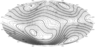Figure 1

Geomagnetic field and its variations. GMF structure is complicated and inhomogeneous, that is why its distribution on the Earth's surface has an anisotropic character. So, induction of GMF on the border of the magnetosphere is $\sim$10.03 mT. It is 20–30 mT at the equator and 60–70 $\mu$T at the poles near the Earth's surface (Figure 1) [Yanovsky 1978].

Here the main field total intensity vector in the point with given spatiotemporal coordinates (latitude, longitude, elevation, year) is defined as a sum of three components:

\begin{eqnarray*} \mathbf{B}_\mathrm{GE} = \mathbf{B}_\mathrm{int} + \mathbf{B}_\mathrm{ext} + \mathbf{B}_\mathrm{tech} \end{eqnarray*}

where $\mathbf{B}_\mathrm{int}$ is a vector of GMF of intraterrestrial sources; $\mathbf{B}_\mathrm{ext}$ is a variable component of GMF, which is caused by influence of external factors and geomagnetic disturbances; $\mathbf{B}_\mathrm{tech}$ is a GMF intensity vector component with technogenic (anthropogenic) origin.

Magnetic field of intraterrestrial sources consists of two fields: electric currents field in the core (the main ("core") field), which is $\sim$98% of the whole field, and rocks magnetism field, which is $\sim$2% of the whole field. Thus, with altitude raise the crust field decreases much quicker, then the main field, and from the altitude $\sim$100 km its value can be neglected.

The calculated or some averaged values of GMF parameters are conditionally accepted as a normal (undisturbed) state of geomagnetosphere. Deviations of actual values of GMF parameters from these normal values are known as geomagnetic variations (GMV) and are estimated by special geomagnetic indices [Zabolotnaya, 2007].

### Overview and Analysis of the Problem Solutions

Information technologies (IT), which provide methods and tools for operating geospatial data, are among the most dynamic technologies. This popularity has two reasons: firstly, 80% of the data has a geospatial reference; secondly, it correlates with fast grow of SQL- and noSQL-technologies.

The Russian standard GOST-R 52438 2005 defines the term "geospatial data" as a data about spatial objects, which are the digital representation of the real objects with information about their location and characteristics. Also the same standard defines geoinformation system (GIS) as a system, which operates with geospatial data (see GOST 25645.126-85 1985, GOST 25645.127-85 1985, and GOST R 52438-2005 2006).

Parameters of space weather, GMF and its variations have a geospatial reference and can be classified as spatial data. So, these data can be operated by the appropriate information technologies, which provide geospatial reference of attributive data, their processing, analysis and visualization.

In spite of wide variety of software solutions and technologies a solution of the problem of digital processing and visualization of parameters of space weather, GMF and GMV is still not or just partially supported by IT and GIS. At the same time an efficiency of this approach to the problem solution is obvious.

Modern tendencies of IT evolution consider web solutions as one of its key aspects. These intentions are referred to the new cloud information space named GeoWeb, where each element has a geographical spatial reference. The basis of GeoWeb is a new special class of GIS called Web GIS, which stands between Web and GIS technologies. GeoWeb platform is supported by international organization Open GIS Consortium (OGS) [Vorobev and Shakirova, 2015b, 2015c, 2015d, 2015e].

The analysis of the problem solutions proved, that information technologies providing calculation, geospatial reference, visualization and automated data processing are poorly developed. One of the known solutions is the information service, which is provided by NOAA and available at http://www.ngdc.noaa.gov/geomag-web. Although the calculation results are acceptable enough, the solution has a lot of disadvantages, such as no visualization tools, low ergonomics and efficiency. The same disadvantages are inherent to other similar services, which are provided by BGS (http://www.geomag.bgs.ac.uk/data_service/models_compa ss/wmm_calc.html), CIRES (http://geomag.org/models/igr fplus-declination.html) and other leading organizations. According to geomagnetic data analysis there is another well-known solution. It is the Interregional Geomagnetic Data Center of the Russian-Ukrainian INTERMAGNET segment, which is operated by the Geophysical Center of the Russian Academy of Sciences ([http://geomag.gcras.ru/index-ru.html]). Geomagnetic data are transmitted from observatories located in Russia and Ukraine [Soloviev, 2011, 2013]. The solution is based on fuzzy logic approach and is intended to real-time recognition of artificial (anthropogenic) disturbances in incoming data. One more key element of the mentioned problem is concerned with a number of heterogeneous data sources, which collect and share historical and current values of parameters of space weather, geomagnetic field and its variations. These are powerful web services provided by INTERMAGNET [Kerridge, 2001], BGS [Dawson, 2013] and other organizations. The main thing here is that all these data sources are not integrated. There are no any tools, which provide to user a single access to space weather and geomagnetic data. In this paper the authors suggest a solution of the problem – the analytical information system (AIS) GEOMAGNET, which is available at https://www.geomagnet.ru. AIS GEOMAGNET provides modeling, monitoring, analytical control, two- and three-dimensional visualizations of space weather, GMF and its variations parameters. With this system a user can get access to data, automatically analyze them and use the results for solving applied problems.

### Conception and Tools of AIS GEOMAGNET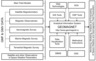Figure 2

Figure 2 represents a conception of the system of monitoring and control of parameters of space weather, GMF and its variations. The conception is based on a paradigm of heterogeneous data sources combination into an integrated information space with platform-independent mechanism of their analytical control and interpretation.

AIS GEOMAGNET provides the main mechanisms of real-time monitoring, modeling, analytical control and forecast of space weather, GMF and its variations parameters (discretization step is 1 minute). AIS GEOMAGNET is based on relevant available data and primarily provides an improvement of informativeness, efficiency, and ergonomics criteria in monitoring and analytical control of space weather, GMF and GMV parameters.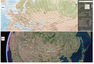Figure 3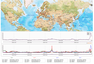Figure 4

AIS GEOMAGNET provides and is based on the following GIS mechanisms:

• direct and reverse geocoding of spatial data;
• interpretation and visualization of spatial data (Figure 3);
• geolocation (the identification of exact users device location);
• processing, visualization, translation and analysis of available magnetic observatories data (with support of INTERMAGNET and Geophysical Center of Russian Academy of Sciences) (Figure 4);
• virtual globe based on WebGL technology (Figure 3b).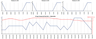Figure 5

AIS GEOMAGNET also supports the following mechanisms of data visualization and interpretation:

• multilayer visualization of spatial data with optional support of user-defined KML/KMZ layers [Vorobev and Shakirova, 2015a];
• visualization of two-dimensional array with dynamic time axis scalability on the basis of web interfaces D3.js – Data-Driven Documents (Figure 4b);
• 2D and 3D visualization of geospatial data with scale-depend detalization level: $n = \log_2 (\log_4 m) + 2,$ where $m$ is a quantity of tiles; $n$ is a discrete scale level;
• forecast (detailed for 3 days and daily-average for 27 days) of space weather and GMF parameters (Figure 5).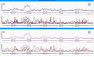Figure 6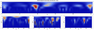Figure 7

AIS GEOMAGNET uses classic mechanisms of digital signal processing (DSP) to provide a set of tools for digital processing of data about space weather, GMF and GMV parameters, such as following:

• linear, nonlinear and adaptive filtering of information signal, which provides noise reduction and signal bandpassing in frequency domain as well as analysis of correlation of adjacent information signals parameters (Figure 6);
• signal analysis in time domain, which provides a calculation of maximal, minimal and average values, variance and standard deviation of information signal;
• spectral and time-frequency analysis, which is implemented by two ways. First way is an analysis of periodogram as an estimation of power spectral density based on calculation of square of data sequence Fourier transformation module with statistical averaging if information signal (1). Second way is an estimation of wavelet scalogram of information signal (Figure 7).

The Sequences 1 and 2 represent main steps of DSP, which are used in AIS GEOMAGNET.

Sequence 1. Main methodic of DSP.

1. Reading the discrete information signal (IS) as JSON/CSV/XML array
2. Detection, analysis and exclusion of missing measurements
3. Calculation and exclusion of IS constant component
4. Caclulation of IS variance
5. Calculation of numerical value of IS frequency threshold detector
6. Calculation of direct Discrete Fourier Transformation (DFT) and periodogram based on Fast Fourier Transform (FFT)
7. Calculation of the biggest IS frequency with power spectral density less than IS frequency threshold detector
8. Calculation of low frequencies digital filter cutoff frequency
9. Preparation of discrete IS (original array) for digital filtering
10. Calculation of direct DFT of IS, which is prepared to filtering
11. Multiplication of Fourier image and transfer function
12. Calculation of reverse DFT of IS on the basis of FFT
13. Preparation of the array to save / output
14. Save / output of the filtered array in JSON/CSV/XML format

Sequence 2. Methodic of information signal time-frequency analysis.

1. Reading of discrete information signal (IS) as array
2. Calculation of IS minimal and maximum values
3. Calculation and exclusion of IS constant component
4. Calculation of IS standard deviation and variance
5. Calculation of IS Fourier transformation and periodogram
6. Calculation of IS frequency threshold detector
7. Calculation of parameters of discretization of IS discrete wavelet transformation
8. Calculation of IS discrete wavelet transformation and scalogram
9. Graphical interpretation of IS discrete wavelet transformation and scalogram

It is necessary to mention, that calculation and exclusion of the constant component on the step 3 is performed according to the following expression:

\begin{equation} \tag*{(1)} x_k^* = x_k - \frac{1}{N} \sum_{k=0}^{N-1} x_k \end{equation}

where $x_k^*$ is $k$-th element of array with constant component excluded from source array $x_k$; $N$ is a length of array.

Next standard deviation and variance of information signal are calculated according to the following expression:

\begin{equation} \tag*{(2)} \sigma^2 = \frac{1}{N-1} \sum_{k=0}^{N-1} (x_k^*)^2 \end{equation}

where $\sigma$ is a standard deviation and $\sigma^2$ is a variance of information signal.

On step 5 (Sequences 1 and 2) a numerical value of information signal frequency threshold detector $l$ is calculated according expression (3):

\begin{equation} \tag*{(3)} l = \frac{\sigma^2 X_q}{N}, \quad \mbox{where } X_q = -\ln (q) \end{equation}

This value is an indicator, that the harmonic exists in information signal not as a component of white noise. Probability of such a statement is $1-q$, where $q\ll 1$ (for example, in this case $q = 0.03$).

Fourier transformation of discrete function with excluded constant component is used on step 6 (Sequences 1 and 2) to calculate an information signal periodogram with expression (4). In case of odd $N$ the upper limit of calculation is $(N+1)/2$:

\begin{equation} \tag*{(4)} D_j = \frac{1}{N^2} \left[ (\mathrm{Re} \, X_j)^2 + (\mathrm{Im} \, X_j)^2 \right] \end{equation}

where $D_j$ is $j$-th element of periodogram; $\mathrm{Re} \, X_j$ and $\mathrm{Im} \, X_j$ are real and imaginary parts of Fourier transformation, which are calculated as follows:

\begin{eqnarray*} X_j = \sum_{k=0}^{N-1} x_k^* e^{-i \frac{2\pi}{N} k_j}, \quad j=0, 1, \dots, N-1 \end{eqnarray*}

So, if $\Delta t$ is a discretization step of source signal, then periodogram counts refer to the frequencies:

\begin{eqnarray*} \nu_j = \Delta \nu \cdot j, \quad j=0, 1, \dots, N/2, \quad \mbox{where } \Delta \nu = \frac{1}{N \Delta t} \end{eqnarray*}

Next, on step 7 (Sequence 2) the discretization parameters for discrete Fourier transformation are calculated. So, for example, rounding up value of maximal scale level $a_{\max}$ is calculated as follows:

\begin{equation} \tag*{(5)} a_{\max} = \log_2 \left( \frac{N}{2} \right) \end{equation}

Next (Sequence 2) a discrete wavelet transformation of information signal is calculated according to the expression (6):

\begin{equation} \tag*{(6)} W(k,a_i,b_j) = \sum_{-N/2}^{N/2} \left[ \frac{1}{\sqrt{a_i}} \cdot x_k^* \cdot \Psi \left( \frac{k-b_j}{a_i} \right) \right] \end{equation}

where $a_i = a_0^m$; $a_0 = 2$; $m = 0,1,\dots,a_{\max}$.

As well as its scalogram:

\begin{equation} \tag*{(7)} S(a_i,b_j) = \left| W(a_i,b_j)^2 \right| \end{equation}

where $\Psi$ is a parent wavelet; $a$ is a scale parameter; $b$ is a shift parameter ($b = -N/2, \dots, N/2$). Steps 9 and 13 (Sequence 1) are concerned with preparations of information signal to digital filtering and a source array to save/output. In both cases the preparation is to multiply each element of the array by value $(-1)^i$, where $i$ is an array element index. This operation provides harmonics count from the origin of coordinate system.

Figure 7 represents an example of the magnetic observatory data wavelet scalogram, which was generated by AIS NET with use of methods of DSP and time-frequency analysis of information signal. Technical realization is based on web graphic APIs D3.js.

AIS GEOMAGNET uses for data interpretation API D3.js. It is a JavaScript library, which provides data analysis and visualization for Web. Because of its platform-independence and web-focus AIS GEOMAGNET is available for the users with any platform and device.

### Conclusion

During the research the authors have suggested and developed a conception of modeling and distant analytical control of space weather, GMF and GMV parameters. The conception is based on a paradigm of heterogeneous data sources (satellite, air, marine, ground observations, and magnetic observatories) combination into integrated information space with platform-independent mechanisms of DSP and data interpretation. The research proved, that a program formalization of the conception as AIS GEOMAGNET reduces the time required for collecting, processing and analyzing heterogeneous data about space weather, GMF and GMV. The AIS provides analytical control and calculation of parameters of main magnetic field with methodical error less than 0.78%. Suggested and developed method of geomagnetic data interpretation [Vorobev and Shakirova, 2015a] on the basis of web GIS and OpenGL provides an increase of responsiveness and efficiency of the appropriate control and research processes.

#### Acknowledgments

The reported study was funded by RFBR according to the research project No. 14-07-00260-a, 15-07-02731-a, and the grant of President of Russian Federation for the young scientists support MK-5340.2015.9, Grant of Republic of Bashkortostan.

### References

Dawson, E., J. Lowndes, P. Reddy (2013), The British Geological Survey's new Geomagnetic Data Web Service, Data Science Journal, 12, p. WDS75–WDS80, doi:10.2481/dsj.wds-010.

Kerridge, D. (2001), INTERMAGNET: Worldwide near-real-time geomagnetic observatory data, ESA Space Weather Workshop, p. 1–4, Eur. Space Res. and Technol. Cent., Noordwijk, Netherlands.

Soloviev, A. (2011), Artificial intelligence in the Earth's magnetic field study and INTERMAGNET Russian Segment, ICSU CODATA Newsletter, 100, p. 4.

Soloviev, A., S. Bogoutdinov, A. Gvishiani, R. Kulchinskiy, J. Zlotnicki (2013), Mathematical Tools for Geomagnetic Data Monitoring and the INTERMAGNET Russian Segment, Data Science Journal, 12, p. WDS114-WDS119, doi:10.2481/dsj.WDS-019.

Thomson, A. (2014), Geomagnetic observatories: monitoring the Earth's magnetic and space weather environment, Weather, 69, no. 9, p. 234–237, doi:10.1002/wea.2329.

Vorobev, A. V., G. R. Shakirova (2015a), An Approach of Processing and Multilayer Visualization of Data With Geospatial Reference: Patent for Invention No 2568274, RosAPO, Moscow.

Vorobev, A. V., G. R. Shakirova (2015b), Applicaton of geobrowsers to 2D/3D-visualisation of geomagnetic field, 15th International Multidisciplinary Scientific GeoConference SGEM 2015 Conference Proceedings, June 18–24, 2015, Vol. 1, p. 479–486, SGEM, Albena, doi:10.5593/SGEM2015/B21/S8.059.

Vorobev, A. V., G. R. Shakirova (2015c), Calculation and analysis of dynamics of main magnetic field parameters for the period 2010–2015, Geoinformatika, no. 1, p. 37–46.

Vorobev, A. V., G. R. Shakirova (2015d), Modeling and 2D/3D-visualization of geomagnetic field and its variations parameters, 15th International Multidisciplinary Scientific GeoConference SGEM 2015 Conference Proceedings, June 18–24, 2015, Vol. 1, p. 35–42, SGEM, Albena.

Vorobev, A. V., G. R. Shakirova (2015e), Web-Based Information System for Modeling and Analysis of Parameters of Geomagnetic Field, Procedia Computer Science, no. 59, p. 73–82, doi:10.1016/j.procs.2015.07.339.

Yanovsky, B. M (1978), The Earth's Magnetism, 590 pp., Izdatel'stvo Leningradskogo universiteta, Leningrad.

Zabolotnaya, N. A. (2007), Geomagnetic activity indices, 84 pp., LKI, Moscow.

Received 20 August 2016; accepted 26 August 2016; published 14 September 2016.Citation: Vorobev A. V., G. R. Vorobeva (2016), Analytical information system for control and spectral analysis of geomagnetic field and space weather parameters, Russ. J. Earth Sci., 16, ES4001, doi:10.2205/2016ES000571.

Generated from LaTeX source by ELXpaper, v.1.5 software package.